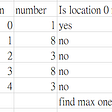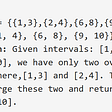# Koko Eating Bananas — Day 106(Python)

Today’s question is a medium-tagged question. Let’s look into the problem statement.

875. Koko Eating Bananas

Koko loves to eat bananas. There are `n` piles of bananas, the `ith` pile has `piles[i]` bananas. The guards have gone and will come back in `h` hours.

Koko can decide her bananas-per-hour eating speed of `k`. Each hour, she chooses some pile of bananas and eats `k` bananas from that pile. If the pile has less than `k` bananas, she eats all of them instead and will not eat any more bananas during this hour.

Koko likes to eat slowly but still wants to finish eating all the bananas before the guards return.

Return the minimum integer `k` such that she can eat all the bananas within `h` hours.

Example 1:

`Input: piles = [3,6,7,11], h = 8Output: 4`

Example 2:

`Input: piles = [30,11,23,4,20], h = 5Output: 30`

Example 3:

`Input: piles = [30,11,23,4,20], h = 6Output: 23`

Constraints:

• `1 <= piles.length <= 10^4`
• `piles.length <= h <= 10^9`
• `1 <= piles[i] <= 10^9`

When I first saw this question, I thought why can’t I just find the maximum bananas from the piles and return it as an answer. But here is the catch, we need to find the “minimum integer `k` such that she can eat all the bananas within `h` hours”.

What would the brute force way be to solve this problem? We can start from 1 banana, check if we can complete the pile before the guard returns. If it’s not possible we increase the count by 1. We keep doing it until we find an integer such that bananas can be completed before the guard returns.

Let us look into the code.

`class Solution:    def minEatingSpeed(self, piles: List[int], h: int) -> int:        current_speed = 1        while(True):            total_time = 0            for pile in piles:                total_time += math.ceil(pile/current_speed)            if total_time <= h:                return current_speed            current_speed += 1`

Complexity Analysis

Time Complexity

The while loop runs until we find the minimum integer k and within the while loop, we have a for loop that runs for all the numbers in piles. Hence the time complexity is O(K*P).

Space Complexity

We are not using any extra space and hence space complexity is O(1).

If we try to run this code in leetcode, we would get Time Limit Exceed Error. How can we optimize it?

If we notice we are running our loop from 1 until we find the minimum integer, and the values are increasing monotonously. Can we use binary search here?

We can consider our start as 1 and our end as the maximum value within the piles array i.e. 10⁹. How would we know the answer? The loop will end when the value of start as well as end is equal and that will be the result or minimum integer that we need to find.

`class Solution:    def minEatingSpeed(self, piles: List[int], h: int) -> int:        low = 1        high = pow(10, 9)        while(low < high):            total_time = 0            mid = (low + high)//2            for pile in piles:                total_time += math.ceil(pile/mid)            if(total_time <= h):                high = mid            else:                low = mid + 1        return low`

Complexity Analysis

Time Complexity

Binary search reduces the time complexity by half each time the while loop run, hence for time complexity for that binary search part is O(logM), where M is the max number. But we need to understand, within while loop we have another for loop which check if the bananas in the piles can be completed in that time. hence the overall time complexity is O(PlogM).

Space Complexity

We are not using any extra space and hence space complexity is O(1).

--

--

--

## More from Annamariya Tharayil

Software Engineer. Find me @ www.linkedin.com/in/annamariya-jt

Love podcasts or audiobooks? Learn on the go with our new app.

## Why I stopped using CSS Frameworks, Should you too?## Bibliography and Citation — Altmetric## WebVR and AFrame Bringing VR to Web at the Virtuleap Hackathon## Mobile App Development: Should I go with Native or nah## Developing and Implementing a Custom Built Locomotion Method## Load-testing Apache Cassandra; Parsing Latency & Throughput Results (Part 1 of 3)## What happens when you type `ls -l *.c` in the shell?## 7 mistakes when using Apache Kafka## Annamariya Tharayil

Software Engineer. Find me @ www.linkedin.com/in/annamariya-jt

## All Elements in Two Binary Search Trees — Day 108(Python)## [LeetCode]#2078. Two Furthest Houses With Different Colors## Weekly Contest 273 Q4, 2122. Recover the Original Array (Hard)## Overlapping Intervals